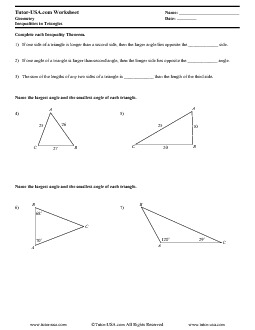Triangle Inequality Theorem
Triangle Inequality Theorem

Triangle Inequality Theorem – Inequalities in Triangles

Worksheet (Geometry)## Samples:

Inequalities in Triangles

Complete each Inequality Theorem.

1) If one side of a triangle is longer than a second side, then the larger angle lies opposite the _____________ side.

2) If one angle of a triangle is larger than second angle, then the longer side lies opposite the _____________ angle.

3) The sum of the lengths of any two sides of a triangle is _____________ than the length of the third side.

4-5) Name the largest angle and the smallest angle of each triangle.

6-7) Name the largest angle and the smallest angle of each triangle. Is it possible for a triangle to have the sides with the lengths indicated? Explain.

8) 3, 7, 8

9) 1, 1, 4

10) 9, 6, 2

11) 3, 4, 5

12) 2.4, 2.9, 5

13) 3, 6, 10

14) Two sides of a triangle have lengths of 10 and 13. The length of the third side can be any number between _____ and ______.

15) Two sides of a triangle have lengths of 5x and 11x. The length of the third side can be any number between _____ and ______.